NEET and AIPMT NEET Chemistry Amines MCQ Questions Solved

An organic compound A on reduction gives compound B which on reaction with chloroform and potassium hydroxide forms C. The compound C on catalytic reduction gives N-methylaniline. The compound A is

(a) nitrobenzene

(b) nitromethane

(c) methylamine

(d) aniline

(a)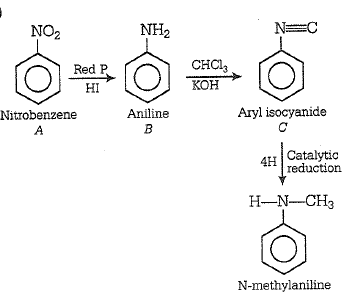Difficulty Level:

• 51%
• 17%
• 15%
• 19%

Acetamide and ethyl amine can be distinguished by reacting with

(a) aq. HCl and heat

(b) aq. NaOH and heat

(c) acidified KMnO4

(d) bromine water

(b) When acetamide is heated with aq. NaOH it forms NH3 gas but ethylamine cannot form NH3.

CH3CONH2 + H2O$\stackrel{\mathrm{NaOH}}{\to }$CH3COONa + NH3

CH3CH2NH2 + H2$\stackrel{\mathrm{NaOH}}{\to }$No reaction

Difficulty Level:

• 13%
• 40%
• 22%
• 27%

Which of the following compound gives dye test

(1) Aniline                (2) Methylamine                  (3) Diphenylamine                  (4)Ethylamine

(1) Basically, all Azo dyes are derivatives of aniline.

Difficulty Level:

• 55%
• 12%
• 29%
• 6%

CH3CH2Cl$\stackrel{\mathrm{NaCN}}{\to }$X$\stackrel{\mathrm{Ni}/{\mathrm{H}}_{2}}{\to }$YZ

In the above reaction sequence, Z is

(a) CH3CH2CH2NHCOCH3

(b) CH3CH2CH2NH2

(c) CH3CH2CH2CONHCH3

(d) CH3CH2CH2CONHCOCH3

(a) CH3CH2Cl $\stackrel{\mathrm{NaCN}}{\to }$CH3-CH2-CN$\stackrel{\mathrm{Ni}/{\mathrm{H}}_{2}}{\to }$CH3-CH2CH2NH2CH3-CH2-CH2-NHCOCH3

X                             Y                                            Z

Difficulty Level:

• 59%
• 17%
• 15%
• 10%

In the reaction,

+2H $\underset{{\mathrm{SnCl}}_{2}}{\overset{\mathrm{HCl}}{\to }}$X Y ,

the term Y is

(a) acetone

(b) ethanamine

(c) acetaldehyde

(d) dimethyl amine

(c)  +2H $\underset{{\mathrm{SnCl}}_{2}}{\overset{\mathrm{HCl}}{\to }}$${\mathrm{CH}}_{3}-\mathrm{CH}=\mathrm{NH}$$\underset{\mathrm{Boil}}{\overset{{\mathrm{H}}_{2}\mathrm{O}}{\to }}$${\mathrm{CH}}_{3}-\mathrm{CH}=\mathrm{O}$

X                               Y

Imide                        Acetaldehyde

So, Y is acetaldehyde.

Difficulty Level:

• 16%
• 36%
• 42%
• 8%

Predict the product,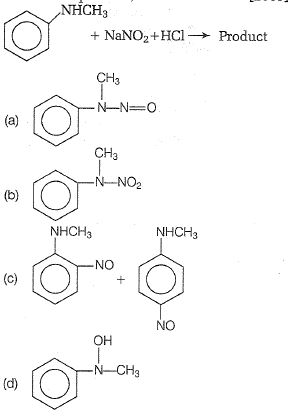(a) Both aliphatic and aromatic secondary amines react with NaNO2 + HCl(HNO2) to form N-nitrosoamines which are insoluble in dilute mineral acids and separate out as neutral yellow oily compounds.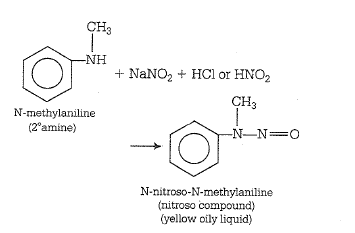Difficulty Level:

• 40%
• 22%
• 30%
• 10%

Aniline in a set of reactions yielded a product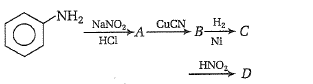The structure of the product D would be

(a) C6H5CH2NH2

(b)C6H5NHCH2CH3

(c)C6H5NHOH

(d)C6H5CH2OH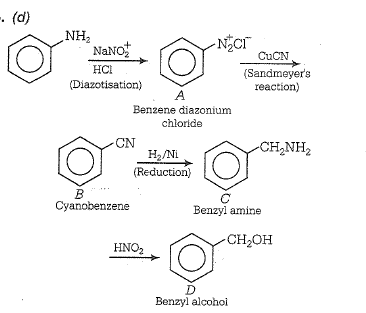Difficulty Level:

• 17%
• 15%
• 26%
• 45%

Intermediates formed during the reaction of RCONH2 with Br2 and KOH are

(a) RCONHBr and RNCO

(b) RNHCOBr and RNCO

(c) RNHBr and RCONHBr

(d) RCONBr2

(a)  The reaction, RCONH2 + Br2 + KOH $\to$RNH2

is known as Hofmann bromamide reaction. The mechanism of this is given as: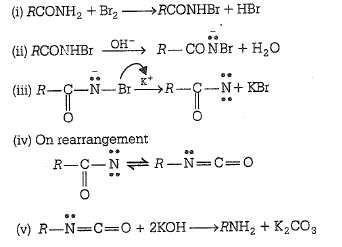Difficulty Level:

• 48%
• 27%
• 21%
• 7%

In a reaction of aniline a colored product C was obtained.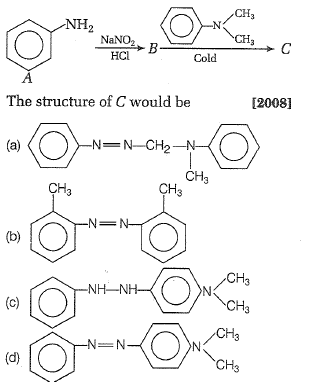(d)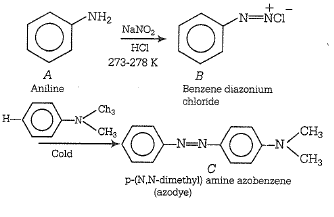Difficulty Level:

• 15%
• 9%
• 12%
• 66%

Which one of the following on reduction with LiAlH4 yields a secondary amine?                   

(a) Methyl isocyanide

(b) Acetamide

(c) Methyl cyanide

(d) Nitroethane

(a) CH3-N$\equiv$C + 4[H] $\stackrel{{\mathrm{LiAlH}}_{4}}{\to }$CH3NHCH3

Dimethylamine

On catalytic reduction or with lithium aluminium hydride (LiAlH4) or with nascent hydrogen, alkyl isocyanide yield 2$°$ amine whereas cyanide gives 1$°$ amine on reduction.

Difficulty Level:

• 71%
• 16%
• 10%
• 5%